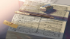Discussion about math, puzzles, games and fun.   Useful symbols: ÷ × ½ √ ∞ ≠ ≤ ≥ ≈ ⇒ ± ∈ Δ θ ∴ ∑ ∫  π  -¹ ² ³ °

You are not logged in.

## #1 2018-02-24 09:32:10

Hannibal lecter
MemberRegistered: 2016-02-11
Posts: 225
Website

### Probability of 3 Heads in a Row

Hi,

what is that " Example: Probability of 3 Heads in a Row "

I saw it in MathIsFun

but it's hard to understand

I knew the law of Probability is  = event / sample space which is mean p(head) = 1/2 = 0.5

so what is the example and what is exactly meaning by " in a row " ?

Example: Probability of 3 Heads in a Row
For each toss of a coin a "Head" has a probability of 0.5:

probability coin hhh = 0.5x0.5x0.5 = 0.125

And so the chance of getting 3 Heads in a row is 0.125

Last edited by Hannibal lecter (2018-02-24 09:32:54)

Wisdom is a tree which grows in the heart and fruits on the tongue

Offline

## #2 2018-02-24 21:17:43

bob bundyRegistered: 2010-06-20
Posts: 8,462

### Re: Probability of 3 Heads in a Row

hi Hannibal lecter

"3 in a row" means you throw a coin once and it's a head, then throw again and it's a head and then again it's a head for a third time.

In a probability question where there is only one event the formula is

probability = (number of ways you get the result you're interested in) / ( total number of things that could happen)

In the case of throwing a coin and getting a head, there are two possibilities (H or T) and one of these gives what you want, so P = 1/2 = 0.5

In situations where more than one event occurs the formula for calculating all the things happening is

probability = (probability of event one) x (probability of event two) x (probability of event three) x ..........

So for three heads that is 0.5 x 0.5 x 0.5

Each coin cannot 'know' what happened with the other coins, so for each the probability is 0.5.

Here's another example:

I pick three cards from a usual pack of 52.  What is the probability of getting three aces ?

prob(first ace) = 4/52

But now that ace has gone so

prob(second ace) = 3/51

and now there are only 2 aces left so

prob(third ace) = 2/50

So Prob(three aces) = 4/52 x 3/51 x 2/50 = 0.000181

Bob

Children are not defined by school ...........The Fonz
You cannot teach a man anything;  you can only help him find it within himself..........Galileo Galilei
Sometimes I deliberately make mistakes, just to test you!  …………….Bob BundyOffline